# THEORY OF PRODUCTION AND COST ANALYSIS - MEFA Notes - JNTU

Posted by Vishnureddy at 11:40 PM1

MANAGERIAL ECONOMICS AND FINANCIAL ANALYSIS
By: B. Mallesham,
Assistant prof,

THEORY OF PRODUCTION AND COST ANALYSIS

THEORY OF PRODUCTION

The production function:-
According to samuelson,”the technical relationship which reveals the maximum amount of output capable of being produced by each and every set of inputs”.
According to Michael R.Baye, “that function which defines the maximum amount of output that can be produced with a given set of inputs.
From the above definitions, it can be seen that:
• The production function is more concerned with physical aspects of productions. It is the concerns of the engineer rather than that of the manager. To know how much be the production with a given set of inputs.
• Production function is defined at a given stage of technical knowledge. It means that if there is any technological break through, there be further jump in the volume of production for the given set of inputs.
• Production function is an engineering relation that expresses the maximum amount of output that can be produced to within a given set of inputs.
• At any given time, the output from a given set of inputs is always fixed.
• Production function enables us to understand how best we can make use of technology to its greatest potential.

Input-output relationship or production function:-
The inputs for any product or service are land, labour, capital, organization & technology.
Q = f (L1, L2, C, OT)
Q = quantity of production
f = function is the type of relationship between inputs and outputs.
A manufacturer has to make a choice of the production function by considering his technical knowledge. He uses lower costs of inputs for a given level of production.
Some factors of production may be important and the relative importance’s of the factors depend upon the final product to be manufactured.

Ex: - in software industry, land is not an input factor as significant as that in case of an agricultural product.
In the case of an agricultural product, increasing the other factors of production can increase the production, but beyond a point increased output can be had only with increased used agricultural land. Investment in land forms a significant portion of the total cost of production for output; where as in the case of the software industry, other factors such as technology, capital, management and others becomes significant. With change in industry and the requirements the production function also needs to be modified to suit to the situation.

Production function with one variable input and laws of returns:-
The law of return states that when at least one factor of production is fixed or factor input is fixed and when all other factors are varied, the total output in the initial stages will increase at an increasing rate, and after reaching certain level of output the total output will increase at declining rate. If variable factor inputs are added further to the fixed factor input, the total output, may decline. This law is of universal nature and it proved to be true in agricultural and industry also. The law of returns is also called the law of variable proportions or the law of diminishing returns.

A B C
Stage I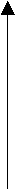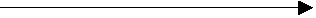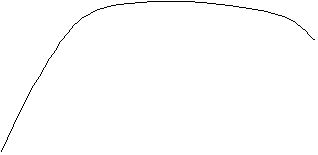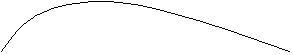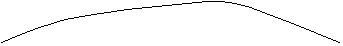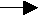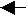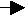Stage II Stage III
Output TP

AP
Units of labour (variable inputs) MP
Total product, average product & marginal product curves.

Outputs with fixed capital and variable labour inputs.
 Units of labour Total product TP Marginal product MP Average product AP Stages 0 1 2 3 4 5 6 7 8 0 10 22 33 40 45 48 48 45 0 10 12 11 7 5 3 0 -3 0 10 11 11 10 9 8 6.85 5.62 Stage I Stage II Stage III

In the short-run, it is assumed that capital is a fixed factor input and labour is variable input. It is also assumed that technology is given and is not going to change. Under such circumstances, the firm starts production with a fixed amount of capital and uses more and more units of labour. In the initial stages, output increases at an increasing rate because capital is grossly under utilized. Productivity will increase up to point ‘A’ where more and more units of labour are increased. After point ‘A’ output increases at a declining rate till it reaches maximum at point ‘C’. After point ‘C’, the total output declines and the margined product of labour is negative. Thus indicates that the additional units of labour are not contributing anything positively to the total output. Even if labour is available free of cost, It is not worth using it.

Production function with two variable inputs and law of returns:-
A production function requires two inputs, capital (C) & labour (L) to produce a given product (Q). This can be more than two inputs in a real life situation, but for a simple analysis, we restrict no. of inputs to two only. The production function based on two inputs can be expressed as,
Q = f (C, 2)
Normally, both capital and labour are required to produce a product. These two inputs can be substituted for each other. Hence the producer may choose any combination of labour and capital that gives the required no. of units of output. For any given level of output, a producer may hire both capital and labour, but he is free to choose any one combination of labour and capital out of several such combinations. The alternative combination of labour and capital yielding a given level of output are such that if the use of one factor input is increased, that of another will decrease and vice versa. However, the units of an input foregone to get one unit of the other input changes, depends upon the degree of substitutability between the two input factors. Based on the techniques or technology used, the degree of substitutability may vary.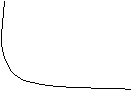XG
Capital F
Increase E
D
C B A IQ 20,000 units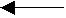O Y
Labour decrease

ISOQUANTS:-
Iso = equal Quant = quantity
Isoquant = the quantities throughout a given Is quant are equal.
Isoquants are also called ‘isoproduct curves’. An Isoquant curve shows various combinations of two input factors such as capital and labour, which yield the same level of output.
An Isoquant curve represents all such combinations which yield equal quantity of output; any or every combination is a good combination for manufacturer. Since he prefers all these combination equally, an Isoquant curve is also called ‘product indifference curve’.

Isoquant yielding 20,000 units ofProductionF
Capital
E
D
C B A

Labour
From the above figure, it shows the different combinations of input factors to yield an output of 20,000 units of output. As the investment goes up, the no. of labourers can be reduced. The combination of ‘A’ shows 1 unit of capital and 20 units of labour to produce say 20,000 units of output. All the above combinations if inputs can be plotted a graph, the locus of all the possible combinations of inputs shows up Isoquant.

Features of an Isoquant:-

Downward sloping: -
These are downward sloping because, if one input increases, the other one reduces. There is no question of increase in both the inputs to yield a given a given output. A degree of substitution is assumed between the factors of production. In other words, an Isoquant cant be increasing, as increase in both the inputs does not yield same level of output remains constant though the use of one of the factors is increasing, which is not true. Isoquants slope from left to right.

Convex to origin: -
Isoquants are convex to the origin. Because the input factors are not perfect substitutes. One input factor can be substituted by other input factor in a diminishing marginal rate. If the input factors were perfect substitutes, the Isoquant would be a falling straight line. When the inputs are used in fixed proportion, and substitution of one input for the other can’t take place, the Isoquant will be shaped.

Do not intersect: -
These two Isoquants don’t intersect, because each of these denote a particular level of output, if the manufacturer wants to operate at a higher level of output, he has to switch over to another Isoquant with a higher level of output & vice versa.

Do not touch the axes: -
The Isoquant touches neither X-axis nor Y-axis, as both inputs are required to produce a given product.

Isoquants where input factors are perfect substitutes…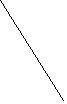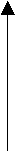Capital
IQ3 = 40,000 units
IQ2 = 30,000 units
IQ1 = 20,000 units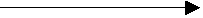O
Labour

Isoquants where inputs factors are not perfect substitutes…Capital IQ3 = 40,000IQ2 = 30,000IQ1 = 20,000O
Labour

Marginal rate of technical substitutes: -
The marginal rate of technical substitution (MRTS) refers to the rate at which one input factor is substituted with the other to attain a given level of output. In other words, the lesser units of one input must be compensated by increasing amounts of another input to produce the same level of output. The below table shows the ratio of MRTS between the two input factors, say capital and labour. 5 units of decrease in labour are compensated by an increase in 1 unit of capital, resulting in a MRTS of 5:1.

Ratio of MRTS between capital and labour:
 Combinations Capital (Rs. In lakh) Labour MRTS A B C D E F 1 2 3 4 5 6 20 15 11 8 6 5 - 5:1 4;1 3:1 2:1 1:1

ISOCOSTS: -
It refers to that the cost curve that represents the combination of inputs that will cost the producer the same amount of money. In other words, each isocost denotes a particular level of total cost for a given level of production. If the level of production changes, the total cost changes and thus the isocost curve moves upwards and vice versa. The below figure shows three downward sloping straight line cost curves (assuming that the input prices are fixed, no quantity discounts are available) each costing Rs. 1.0 lakhs, Rs. 1.5 lakhs & Rs. 2.0 lakhs for the output levels of 20,000 , 30,000 and 40,000 units. (The total cost, as represented by each cost curve is calculated by multiplying the quantity of each input factor with its respective price). Isocosts farther from the origin, for given input costs, are associated with higher costs. Any change in input prices changes the slope of isocost lines.

X
IC3 = 2.0

Capital IC2 = 1.5
In
Lakhs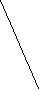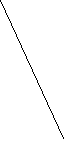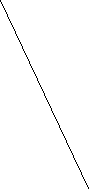IC1 = 1.0

O labour Y
Isocosts each representing different levels of total costs.

Least cost combination of inputs:
The manufacturer has to produce at lower costs to attain higher profits. The Isocosts and Isoquants can be used to determine the input usage that minimizes the cost of production.
Where the slope of Isoquant is equal to that of isocost, there lies the lowest point of cost of production. This can be observed by superimposing the isocosts on isoproduct curves, from the below figure, it is evident that the producer can with a total outlay of Rs. 1.5 lakh, reach the highest Isoquant curve which is IQ2, of he wants to reach IQ3, he has to bring additional resources, which is, let us assume, not possible he cant compromise with IQ!, as it means lower output. There is no other input combination on IQ2 other than point Q, which is cheaper than Rs. 1.5 lakh, so the obvious choice for the producer is Q combination of inputs only on IQ2.
The points of tangency P, Q & R on each of the Isoquant curves represent the least cost combination of inputs, yielding maximum level of output any output lower or higher than this will result in higher cost of production.

X least count combinations of input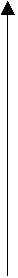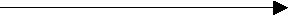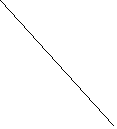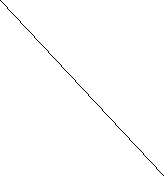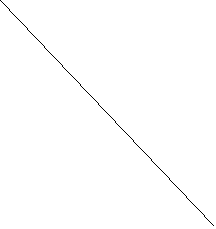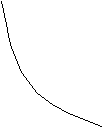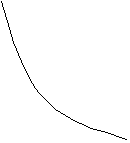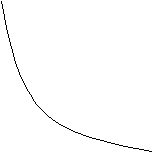IC3 = 2.0

IC2 = 1.5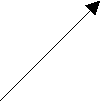Expansion path
IC1 = 1.0

Capital
IQ3 = 40,000 units
IQ2 = 30,000 units

IQ1 = 20,000 units

O labour Y

Production function where input factors are multiple: -
Production function in a two input factor setting may look too easy to be useful. It has been a valuable tool for the managers to decide what combination of inputs yields a given output at the lowest costs. In case of multiple inputs factors, quantitative techniques such as optimization techniques & linear programming provide solution. Computers have further simplified function in a multiple input factor setting.

COBB- DOUGLAS production function:-
Cobb & Douglas put forth a production function relating output in American manufacturing industries from 1899 to 1922 to labour & capital inputs. They used for the following formula:
Formula: P = b L^a C^(1-a).
Where, P is total output.
L is the index of employment of labour in manufacturing.
C is the index of fixed capital in manufacturing.
The exponents ‘a’ and ‘1-a’ are the elasticities of production. These measure the percentage response of output to percentage charges in labour and capital respectively.
The function estimated for the USA by Cobb & Douglas is
P = 1.01 L^0.75 C^0.25.
The production function shows that one percent change in labour input capital remaining the same is associated with a 0.75 percentage change in output. Similarly one percentage change in capital, labour remaining the same, is associated with a 0.25 percent change in output. The coefficient of determination (R^2) means that 94.3 of the variations on the dependent variable (P) were accounted for by the variations in the constant returns to scale which means that there are no economics or diseconomics of large scale of production. On an average, large or small scale plants are considered equally profitable in the US manufacturing industry. On the assumption that the average and marginal production costs were constant.
Though cobb-douglas production function was based on macro-level study, it has been very useful for interpreting economic results. later investigation revealed that the sum of the exponents might be very slightly larger than unity, which implies decreasing costs. But the difference was so marginal that constant costs would seem to be safe assumption for all practical purposes.
Returns to scale & returns to factors: -
Returns to scale refers to the returns enjoyed by the firm as a result of change in all the inputs. It explains the behaviour of the returns when the inputs are changed simultaneously. The returns to scale are governed by laws of returns to scale.

Law of returns to scale: -
There are three laws of returns governing production function.
1. Law of increasing returns to scale.
2. Law of constant returns to scale.
3. Law of decreasing returns to scale.

Law of increasing returns to scale: -
This law states that the volume of output keeps on increasing with every increase in the inputs. Where a given increase in inputs leads to a more than proportional increase in the output, the law of increasing returns to scales is said to operate. We can introduce division of labour and other technological means to increase production. Hence the total product increases at an increasing rate.

Law of constant returns to scale: -
When the scope for division of labour gets restricted, the rate of increase in the total output remains constant, the law of constant returns to scale is said to operate. This law states that the rate of increase/decrease in volume of output is same to that of rate of increase/decrease in inputs.

Law of decreasing returns to scale: -
Where the proportionate increase in the inputs does not lead to equivalent increase in output, the output increases at a decreasing rate, the law of decreasing returns to scale is said to operate. This results in higher average cost per unit.
These laws can be illustrated with an example of agricultural land. Take one acre land, if you fill the land well with adequate bags of fertilizers and sow good quality seed, the volume of output increases. The following table illustrates:

 Capital (in units) Labour (in units) % increase in both input Output (in units) % increase in output Law of applicable. 1 2 4 8 3 6 12 24 - 100 100 100 - 120 240 360 - 140 100 50 - Law of increasing returns to scale. Law of constant returns to scale. Law of decreasing returns to scale.
From the above table, it is clear that with 1 unit of capital and 3 units of labour, the firm produces 50 units of output. When the inputs are doubled two units of capital and six units of labour, the output has gone up to 120 units. (From 50 units to 120 units). Thus when inputs are increased by 100 percent, the output has increased by 140% that is, output has increased by more than double. This governed by law of increasing returns to scale.
When the inputs are further doubled that is to 4 units of capital and 12 units of labour the output has gone up to 240 units (from 120 units to 240 units). Thus when inputs are increased by 100%, the output has increased by 100%. That is output also has doubled. This is governed by law of constant returns to scale, when the inputs are further doubled, that is, to 8 units of capital and 24 units of labour, the output has gone up to 360 units. (From 240 units to 360 units). Thus when inputs are increased by 100%, which has increased only 50%. This is governed by law of decreasing returns to scale.

Returns to factors: -
It is also called factor productivities. Productivity is the ratio of output to the input. Factor productivity refers to the short-run relationship of input and output. The productivity of one unit of a factor of production will be equal to the output it can generate. The productivity of a particular factor is measured with the assumption that the other factors are not changed or remain unchanged. Only that particular factor under study is changed.
Returns to factors refer to the output or return generated as a result of change in one or more factors, keeping the other factors unchanged. Given a percentage of increase or decrease in a particular factor such as labour,

The change in productivity can be measured in terms of:

Total productivity: -
The total output generated at varied levels of input of a particular factor (while other factors remain constant), it is called total physical product.

Average productivity: -
The total physical product divided by the number units of that particular factor used yields average productivity.

Marginal productivity: -
The marginal physical product is the additional output generated by adding an additional unit of the factor under study, keeping the other factors constant.

The total physical product increases along with an increase in the inputs. However the rate of increase is varied, not constant. The total physical product at first increases at an increasing returns to scale, and later its rate of increasing declines because of the law of decreasing returns to scale.

Economies and diseconomies of scale: -
Alfred marshal divided the economies of scale into 2 groups
1. Internal.
2. External.

Internal economies:-
Internal economies refer to the economies in production costs which accrue to the firm alone when it expands its output. The internal economies occur as a result of increase in the scale of production.
These are types of internal economies:
1. Marginal economies.
2. Commercial economies.
3. Financial economies.
4. Technical economies.
5. Marketing economies.
6. Risk-bearing economies.
7. Indivisibilities and automated machinery.
8. Economies of larger dimention.
9. Economies of research & research.

Marginal economies: -
An the firm expands, the firm needs qualified managerial personal to handle each of its functions; - marketing, finance, production, human resources and others in a professional way. Functional specialization ensures minimum wastage & lowers the cost of production in the long-run.

Commercial economies: -
The transactions of buying and selling raw materials and other operating supplies such as spares and so on will be rapid and the volume of each transaction also grows as the firm grows. There could be cheaper savings in the procurement, transaction & storage costs. This will lead to lower costs and increased profits.

Financial economies: -
There could be cheaper credit facilities from the financial institutions to meet the capital expenditure or working capital requirements. A larger firm has larger assets to give security to the financial institution which can consider reducing the rate of interest on the loans.

Technical economies: -
Increase in the scale of production follows when there is sophisticated technology available and the firm is in a position to hire qualified technical man power to make use of there could be substantial savings in the hiring of man power due to larger investments in the technology. This lowers the cost per unit substantially.

Marketing economies: -
As the firm grows larger and larger. It can afford to maintain a full fledged marketing department independently to handle the issues related to design of customer surveys, advertising materials, and promotion campaign handling of sales and marketing staff, renting of hoardings, launching a new product and so on. In the normal course, the firm spends large amounts on issues in marketing and is still not sure of the results because these are handled by different outride groups, on whom there is little control for the firm.

Risk bearing economies: -
As there is growth in the size of the firm, there is increase in the risk also. Sharing the risk with the insurance companies is the risk priority for any firm. The firm can insure its machinery and other assets against the hazards of fire, theft & other risks, the large firms can spread their risk so that they do not keep all their eggs in one basket. They purchase raw material from different sources.

Indivisibilities and automated machinery: -
To manufacture goods, a plant of certain maximum capacity is required whether the firm would like to produce and sell at the full capacity or not, because the production is lesser, the firm can’t hire half the manager or half the telephone. The minimum quality can be produced in the firm. A firm producing below such minimum quantity will have to bear higher costs.

Economies of larger dimention: -
Large scale production is required to take advantage of bigger size plant and equipment. For example, the cost of a 1,00,000 units capacity plant will not be double that of 50,000 units capacity plant like wise, the cost of a 10,000 tonnes oil tanker will not be double that of a 5,000 tonnes oil tanker. Engineering go by what is called two third (2/3) rule. When the volume is increased by 100%, the material required will increase by 100%, the material requires will increase only by two-thirds.
Technical economies are available only from large size, improved methods of production processes & when the products are standardized.

Economies of research & development: -
Large organizations such as Dr. Reddy’s labs, Hindustan lever spend heavily on research and development and bring out several innovative products, only such firms with strong research & development base can cope with competition globally.

External economies: -
These refer to all the firms in the industry because of growth of the industry as a whole or because of growth of auxillary industries. External economies benefit all the firms in the industry as the industry expands. This will lead to lowering the cost of production and thereby increasing the profitability.

The external economies are grouped to 3 types.
1. Economies of concentration.
2. Economies of research and development.
3. Economies of welfare.

Economies of concentration: -
All the firms are located one place. Than there is better infrastructure in terms of
• Transportation facilities such as railway lines.
• Banking & communication facilities.
• Availability of skilled labour.

Economies of research and development: -
All the firms can pool resources together to finance research and development activities and thus share the benefits of research. There could be a common facility to share journals, news papers & other valuable reference material of common interest.

Economies of welfare: -
There could be common facilities such as
• Canteen.
• Industrial housing.
• Community halls.
• Schools and colleges.
• Hospitals.
Those are commonly used by employees

Diseconomies: -
Diseconomies are mostly managerial in nature. Problems of planning, coordination communication and control may become increasingly complex as the firm grows in size resulting in increasing average cost per unit. Sometimes the firm may also collapse.
Diseconomies of scale are said to result when an increase in the scale of production leads to a higher cost per unit.
Ex:- All inputs an increase by 10%, but production increased by 5%, it is called diseconomies.
As the firm grows layer, it may need to seek permission to operate in foreign markets. Any degree of bureairatic inefficiency will affect the firm’s profitability and this leads to diseconomies of scale.
When a firm is not in a position to respond to the business environment in which operates diseconomies are bound to result in. modern organizations have started delaying their organizations to make them flat and lean to overcome the problems of diseconomies.

Tags:

## Search Entire Site

Custom Search